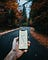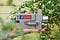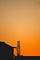# Introduction

Most people are aware of the shortest path problem, but their familiarity with it begins and ends with considering the shortest path between two points, A and B. The shortest path problem is the problem of finding a path between two vertices or nodes in a graph such that the sum of the weights of its constituent edges is minimized. The Shortest path can help us to analyze the information spreading performance and research the latent relationship in the weighted social network. The single-source shortest path algorithm) is also known Bellman-Ford algorithm is used to find the minimum distance from the source vertex to any other vertex. At first, it finds those distances which have only one edge in the path.

Blind search algorithms such as breadth-first and depth-first exhaust all possibilities; starting from the given node, they iterate over all possible paths until they reach the goal node. These algorithms run in O(V+E), or linear time, where V is the number of vertices, and E is the number of edges between vertices.

Algorithms such as Greedy, Dijkstra’s algorithm, and A* eliminate paths either using educated guesses(heuristics) or distance from source to node V to find the optimal path. By eliminating impossible paths, these algorithms can achieve time complexities as low as O (E log(V)).

The Best Shortest Path Algorithms

Dijkstra’s Algorithm.

Bellman-Ford Algorithm.

Floyd-Warshall Algorithm.

Johnson’s Algorithm.

Final Note.

Dijkstra’s Algorithm.

Dijkstra’s Algorithm starts at the node that you choose (the source node), and it analyses the graph to find the shortest path between that node and all the other nodes in the graph. The algorithm keeps track of the currently known shortest distance from each node to the source node and it updates these values if it finds a shorter path. Once the algorithm has found the shortest path between the source node and another node, that node is marked as “visited” and added to the path. The process continues until all the nodes in the graph have been added to the path. This way, we have a path that connects the source node to all other nodes following the shortest path possible to reach each node.

Dijkstra’s Algorithm stands out from the rest due to its ability to find the shortest path from one node to every other node within the same graph data structure. This means, that rather than just finding the shortest path from the starting node to another specific node, the algorithm works to find the shortest path to every single reachable node — provided the graph does not change.

Example:

Dijkstra’s algorithm will assign some initial distance values and will try to improve them step by step. … For example, if the current node A is marked with 6, and the edge connecting it with a neighbor B has a length of 2, then the distance to B through A will be 6 + 2 = 8.

Example of Dijkstra’s Algorithm

The algorithm will generate the shortest path from node 0 to all the other nodes in the graph. For this graph, we will assume that the weight of the edges represents the distance between two nodes. We will have the shortest path from node 0 to node 1, from node 0 to node 2, from node 0 to node 3, and so on for every node in the graph.

# Real-world use cases:

Digital Mapping Services in Google MapsPhoto by Tamas Tuzes-Katai on Unsplash

Social Networking ApplicationsPhoto by Sticker Mule on Unsplash

Telephone NetworkPhoto by Adib Hussain on Unsplash

IP routing to find Open shortest Path First.

Robotic Path

Bellman-Ford Algorithm

Like Dijkstra’s algorithm, the Bellman-Ford algorithm works to find the shortest path between a given node and all other nodes in the graph.

Though it is slower than the former, Bellman-Ford makes up for its disadvantage with its versatility. Unlike Dijkstra’s algorithm, Bellman-Ford is capable of handling graphs in which some of the edge weights are negative. It is important to note that if there is a negative cycle — in which the edges sum to a negative value — in the graph, then there is no shortest or cheapest path. Meaning the algorithm is prevented from being able to find the correct route since it terminates on a negative cycle. Bellman-Ford can detect negative cycles and report on their existence.

Floyd-Warshall Algorithm

The Floyd-Warshall stands out in that unlike the previous two algorithms it is not a single-source algorithm. Meaning, it calculates the shortest distance between every pair of nodes in the graph, rather than only calculating from a single node. It works by breaking the main problem into smaller ones, then combines the answers to solve the main shortest path issue. Floyd-Warshall is extremely useful when it comes to generating routes for multi-stop trips as it calculates the shortest path between all the relevant nodes.

Floyd Warshal Algorithm is used to find the shortest distances in a graph. Dynamic Programming is the algorithm design technique that finds the shortest distances between all the pairs of vertices in a graph. Dynamic Programming Technique uses Floyd Warshal Algorithm, where all pairs of shortest distance in a graph could be found.

Johnson’s Algorithm

Johnson’s algorithm works best with sparse graphs — one with fewer edges, as it is runtime depends on the number of edges. So, the fewer edges, the faster it will generate a route. This algorithm varies from the rest as it relies on two other algorithms to determine the shortest path.

First, it uses Bellman-Ford to detect negative cycles and eliminate any negative edges. Then, with this new graph, it relies on Dijkstra’s algorithm to calculate the shortest paths in the original graph that was inputted. Johnson Algorithm is used to find the shortest paths between every pair of vertices in each weighted directed graph and here weights may be negative. Johnson Algorithm uses both Dijkstra and Bellman-Ford algorithms as subroutines.

Final Note

Often, the best algorithm to use will not be left up to you to decide, rather it will be dependent upon the type of graph you are using and the shortest path problem that is being solved. with negative weight edges, you would turn to Bellman-Ford, whereas for sparse graphs with no negative edges you would turn to Dijkstra’s algorithm.

BFS, DFS, Dijkstra, Greedy, & A* Algorithms. These algorithms are used to search the tree and find the shortest path from starting node to the goal node in the tree.

…As we discussed Dijkstra Algorithm before so, there will not be discussed now in this column.

Breadth-First Search (BFS)

It starts at the root and explores all its children in the next level(neighbors) before moving to each of the root children, and then, it explores the children of the root children, and so on. The algorithm uses a queue to perform the BFS.

A- (B, C)- (D, E, F)-G

Depth-First Search (DFS)

It starts at the root and explores one of its children’s subtree, and then moves to the next child’s subtree, and so on. It uses stack, or recursion to perform the DFS.

A-B-D-C-F-G

Greedy

Greedy is an algorithm that makes a choice based on educated guesses(heuristics) at each stage. The node with the shortest heuristic distance from the goal node will be explored next.

Given the heuristic distance of A, B, C, D, E, & F to goal node equals 8, 8,6, 5, 1, & 4, respectively.

A-C-F-G

A*(A star)

A* is a combination of Dijkstra and Greedy. It uses the distance from the root node plus heuristics distance to the goal. The algorithm terminates when we find the goal node.

A-C-D-E-G